Courses

# Test: Signals And Systems & Microprocessors - 1

## 15 Questions MCQ Test GATE ECE (Electronics) 2022 Mock Test Series | Test: Signals And Systems & Microprocessors - 1

Description
This mock test of Test: Signals And Systems & Microprocessors - 1 for Electronics and Communication Engineering (ECE) helps you for every Electronics and Communication Engineering (ECE) entrance exam. This contains 15 Multiple Choice Questions for Electronics and Communication Engineering (ECE) Test: Signals And Systems & Microprocessors - 1 (mcq) to study with solutions a complete question bank. The solved questions answers in this Test: Signals And Systems & Microprocessors - 1 quiz give you a good mix of easy questions and tough questions. Electronics and Communication Engineering (ECE) students definitely take this Test: Signals And Systems & Microprocessors - 1 exercise for a better result in the exam. You can find other Test: Signals And Systems & Microprocessors - 1 extra questions, long questions & short questions for Electronics and Communication Engineering (ECE) on EduRev as well by searching above.
QUESTION: 1

### In a continuous time system x(t) and y(t) denotes the input and output respectively then which of the following corresponds to a casual system?

Solution: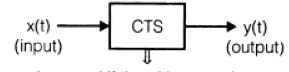is casual if the y(I) at any the
depends only on presarit of past or both
present and past values of xit).

So, here y(t) = + 4) x(t -1) is only a casual system.

QUESTION: 2

### If f(x) =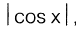, for Fourier series of x(t) in the interval (-π, π), the value of ao and a1 respectively is

Solution:

Since,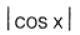is an even function_

So.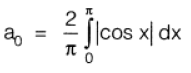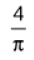and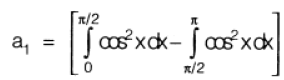= 0

*Answer can only contain numeric values
QUESTION: 3

### The fundamental period of signal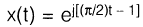Solution: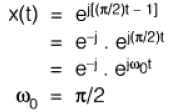Here,

So, fundamental period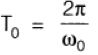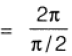= 0

QUESTION: 4

The Laplace transform of a continuous-time signal x(t) is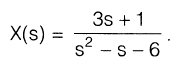If the Fourier transform of this signal exists, then x(t) is

Solution: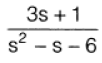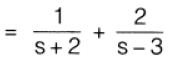If the Fourier transform of the signal exiss, then ROC must be-2 < Re(s) < 3. Therefore, x(t) e 2t u(t) — 2e31 u(-t).

QUESTION: 5

The ROC for the Laplace transform of signal

x(t) = e-2t {u(t) — u(t — 5)] is given by

Solution: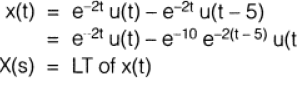∴​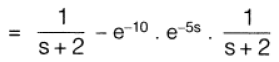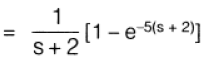for, Re(s) > -2

QUESTION: 6

Under what condition the energy of signal g1(t) and g2(t) equals to E91 + Eg2

Solution: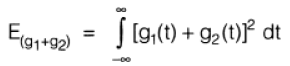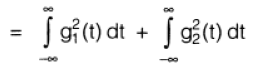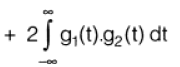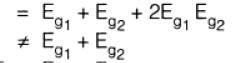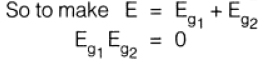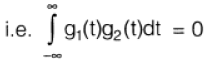QUESTION: 7

The signal V(t) = cos 5 πt + 0.5 cos 10 πt,is instantaneously sampled. The interval between the samples is 'TS'. Then the maximum allowable value for 'Ts' is

Solution:

(c)

Frequencies in the given signals are 2 πf = 5 π and 2 πf = 10 π i.e. 2.5 Hz and 5 Hz.

∴. Sampling frequency

= fs

= 2 fm

= 10 Hz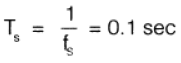QUESTION: 8

Inverse Laplace transform of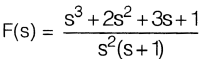Solution: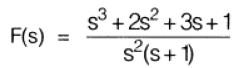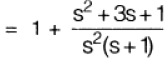Solving by use of partial fraction;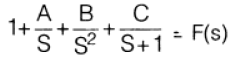We get,          A = 2,

B = 1

and      and      C = --1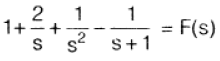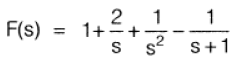∴  f(t)  Inverse laplace of F(s)

f(t)  =  δ(t) + 2 + t - e-t

QUESTION: 9

Match List-I (Type of Addressing Modes)

with List-II (Instructions set) and select the correct answer using the codes given below the lists:

List-I

3. Register indirect mode

List-II

1. MOV A, M

2. MOV A, B

3. CMA

4. STA 2700H

Codes: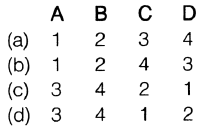Solution:
QUESTION: 10

Consider the following statements:

1. Programme Status Word (PSW) = Accumulator + Flag Register + Program Counter
2. Program counter is a 16-bit register.
3. Stack pointer operates as last in last out.
4. "TRAP" is both level and edge sensitive. Which of the statements are false?
Solution:

PSW = Accumulator Flag Registor only

and SP operates as LIFO (Last in First out).

QUESTION: 11

In priority orders, the interrupts in microprocessor are as

Solution:

In priority order, interrupts are as,

TRAP > RST7 .5 > RST6_5 HST5.5> INTR.

QUESTION: 12

A memory chip of size 4 k byte has starting location 2000H. The last location of memory chip is given by

Solution:

Total number of memory locations

= 22 x 210

212

Since in itia memory location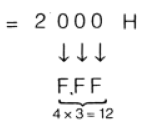QUESTION: 13

The 8085 assembly language instruction that stores the content of H and L register into the memory locations 2050H and 2051H, respectively is

Solution:
QUESTION: 14

The following program is run on an 8085 microprocessor:

(in hex)

3000                                LXI SP, 4000

3003                                 PUSH H

3004                                 PUSH D

3005                                  CALL 3050

3008                                  POP H

3009                                   HLT

At the completion of execution of the program, the program counter of 8085 and stack pointer contains

Solution:

(c)

3000            SP → 4 4000

3003             SP → SP decremented                        by 2      i_e_ 3FFE

3004             SP SP decremented                        by 2  3FFC

3005          PC 3050

SP  3FFC

PC 3050

QUESTION: 15

15 8 memory chips of 64 x 4 bit size have address buses connected together. The resultant memory is

Solution: